Latest Banking jobs   »   Reasoning Ability Quiz For SBI Clerk...

# Reasoning Ability Quiz For SBI Clerk Prelims 2021- 7th June

Directions (1-5): Study the information carefully and answer the questions given below.
Seven persons are sitting around a circular table. Some of them facing towards the center and some of them facing outside the center. J and K sit immediate left to each other. M sits second to the right of K. Two persons sits between S and M. P sits third to the left of L, who is not immediate neighbor of K. Immediate neighbor of L face opposite direction to L. Y face opposite direction to P. Immediate neighbor of Y face opposite direction to each other. Not more than three persons face towards the center.

Q1. Who among the following person sit second to the right of Y?
(a) J
(b) P
(c) S
(d) K
(e) None of these

Q2. How many persons face opposite to the center?
(a) Three
(b) One
(c) Five
(d) Four
(e) None

Q3. How many persons are sitting between S and P, when counted left of P?
(a) Four
(b) Two
(c) Three
(d) More than four
(e) None

Q4. Four of the following five are alike in certain way based from a group, find the one that does not belong to that group?
(a) Y-K
(b) K-P
(c) M-P
(d) L-S
(e) J-L

Q5. What is the position of Y with respect to K?
(a) Fourth to the right
(b) Immediate left
(c) Second to the left
(d) Third to the right
(e) None of these

Directions (6-10): Study the information carefully and answer the questions given below.
Point A is 8m west of point T. Point M is 10m north of point T. Point K is 4m south of point G. Point H is 7m east of point U. Point H is 11m west of point K. point H is 12m south of point M.

Q6. What is the shortest distance between H and G?
(a) √137m
(b) 13m
(c) 14m
(d) √143 m
(e) None of these

Q7. Point T is in which direction with respect to G?
(a) South-west
(b) North-east
(c) West
(d) North
(e) South-West

Q8. What is the shortest distance between Point T and Point U?
(a) 7m
(b) √53m
(c) 8m
(d) √43m
(e) None of these

Q9. What is the direction of T with respect of U?
(a) North-west
(b) South
(c) North
(d) East
(e) North-east

Q10. If point N is 11m west of point G In which direction of point N with respect to Point H?
(a) North-west
(b) East
(c) West
(d) South
(e) None of these

Directions (11-15): The following questions are based on the five three digits numbers given below:

583    659    427    361    416

Q11. If in each number, all the three digits are arranged in ascending order within the number, which of the following will be the fourth lowest number?
(a) 583
(b) 659
(c) 427
(d) 361
(e) 416

Q12. If first digit will be changed (replace) with third digit, then second with first digit and after that third digit with the second digit in each number, then which number will be the lowest?
(a) 583
(b) 659
(c) 427
(d) 361
(e) 416

Q13. If the position of first and third digits of the numbers are interchanged with each number then how many numbers thus formed will be completely divisible by 2?
(a) None
(b) Three
(c) More than three
(d) One
(e) Two

Q14. What will be result if first digit of second highest number is divided by second digit of second lowest number?
(a) 4
(b) 5
(c) 7
(d) 2
(e) 1

Q15. If in each number, both first and second digits are added and then third digit will be subtracted from that addition then which of the following will be the highest resultant?
(a) 4
(b) 2
(c) 1
(d) 8
(e) 10

Practice More Questions of Reasoning for Competitive Exams:

###### SBI Clerk Study Plan 2021

Solutions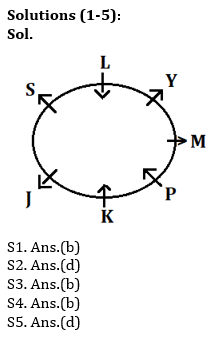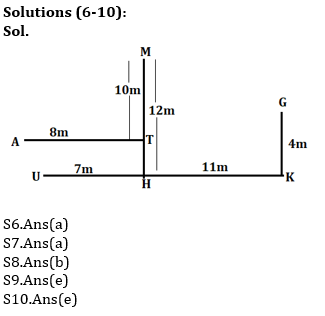S11. Ans.(a)
Sol. After arranging all the digits in ascending order within each number–
358 569 247 136 146
So, the fourth lowest number will be 583.

S12. Ans.(e)

S13. Ans.(b)
Sol. After interchanging first and third digits according to the given condition in the question—
385 956 724 163 614
So number completely divisible by 2 will be three.

S14. Ans.(b)
Sol. First digit of second highest number is 5 and second digit of the second lowest number is 1. So, the resultant will be 5.

S15. Ans.(e)#### Congratulations!

Incorrect details? Fill the form again here

•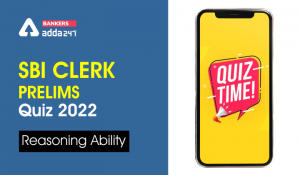Reasoning Ability Quiz For SBI Clerk Pre...
•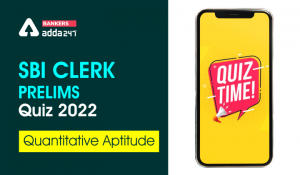Quantitative Aptitude Quiz For SBI Clerk...
•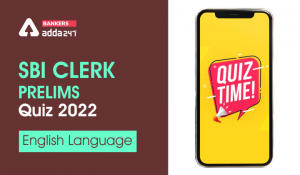English Quizzes For SBI Clerk Prelims 20...
•Quantitative Aptitude Quiz For SBI Clerk...
•English Quizzes For SBI Clerk Prelims 20...
•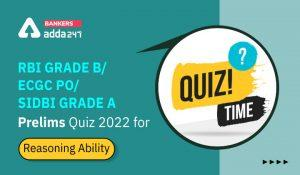Reasoning Ability Quiz For RBI Grade B/ ...